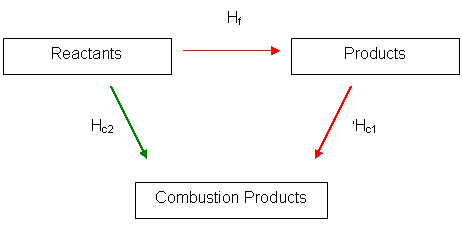# How can the standard enthalpy of formation of CO be calculated?

Apr 17, 2015

You can calculate it by constructing an energy cycle:Enthalpies of formation are often difficult to measure directly. It is a lot easier to measure the enthalpy of combustion of the compound and the elements from which it is made.

Hess' Law states that the total enthalpy change of a reaction is independent of the route taken.

From the energy cycle you can see that the enthalpy change of the $\textcolor{red}{\text{RED}}$ route is equal to the enthalpy change of the $\textcolor{g r e e n}{\text{GREEN}}$ route.

This is because their arrows start and finish in the same place.

We need to find $\textcolor{red}{\Delta {H}_{f}}$ for:

${C}_{\left(s\right)} + \frac{1}{2} {O}_{2 \left(g\right)} \rightarrow C {O}_{\left(g\right)}$

You use :

${C}_{\left(s\right)} + {O}_{2 \left(g\right)} \rightarrow C {O}_{2 \left(g\right)}$ color(green)(DeltaH_(c2)=-394"kJ"

$C {O}_{\left(g\right)} + \frac{1}{2} {O}_{2 \left(g\right)} \rightarrow C {O}_{2 \left(g\right)}$ color(red)(DeltaH_(c1)=-283"kJ"

Applying Hess' Law : $\textcolor{red}{\text{Red route")=color(green)("Green route}}$$\Rightarrow$

$\textcolor{red}{\Delta {H}_{f} - 283}$= $\textcolor{g r e e n}{- 394}$

$\textcolor{red}{\Delta {H}_{f}} = - 111.0 \text{kJ/mol}$